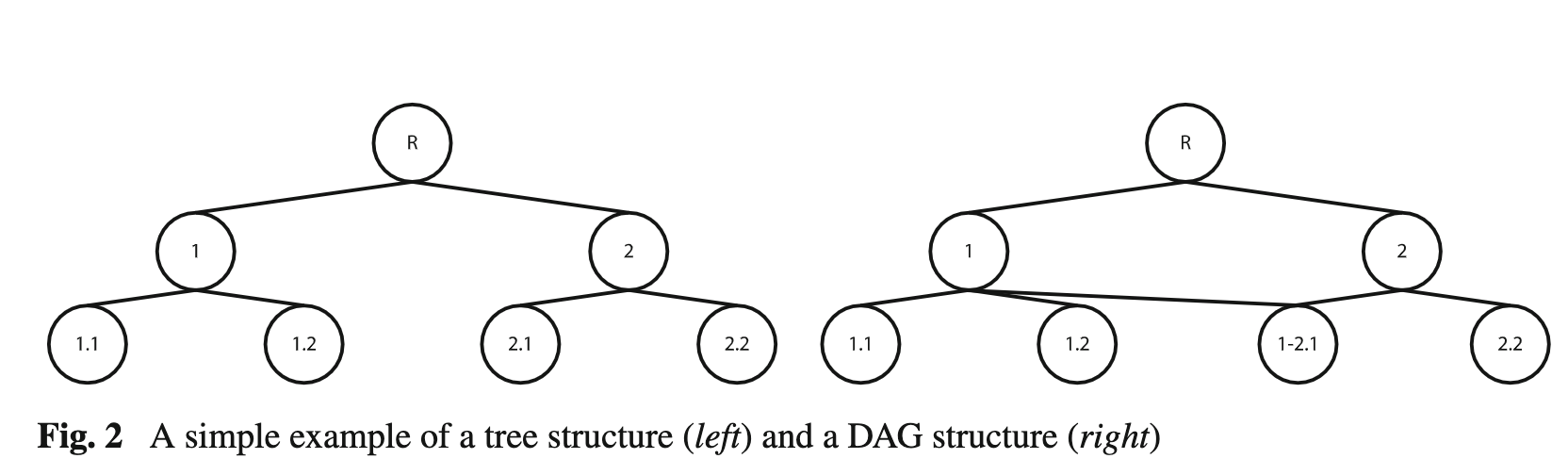## Hierarchical Classification Problem

Hierarchical classification labels involves hierarchical class labels. The hierarchical class labels maybe predefined or inferred. 1

### Class Taxonomy

A hierarchical classification problem comes with a class taxonomy.

• “IS-A” operator: $\prec$,
• “IS-NOT-A” operator: $\nprec$

A IS-A relationship of the labels $c_a$ class set $C$ is

• one root $R$ in the tree,
• asymmetric, i.e., $c_i \prec c_j$ and $c_j\prec c_i$ can not be both true,
• anti-reflexive, i.e., $c_i \nprec c_i$,
• transitive, i.e., $c_i \prec c_j$ and $c_j\prec c_k$ $\Rightarrow$ $c_i \prec c_k$.

There are different representations of the hierarchical taxonomies.Figure 2 in Silla2011, showing the difference between tree taxonomy and DAG taxonomy.
• A classifier doesn’t necessarily classify the labels to the leaves on the tree all the time.
• A classifier can utilize the tree of DAG differently:
• top-down: local classifiers that only utilizes partial hierarchical information, e.g., the prediction of the children will use the prediction of the parent as input; ;
• big-bang: global classifiers with each classifier utilizes the whole paths;
• flat: classifiers ignores the hierarchical relations and predicting the leaves.

## Flat Classification

Classify the leaves and forget about the hierarchies.

1. The hierarchical information is not being used.

## Global Classifiers

Also called Big-Bang approach, golbal classifiers can utilize

1. Clusters in the taxnomony,
2. Multi-label classification with full paths,
3. Revise models, e.g., loss function calculation to include hierarchical information.

Planted: by ;

wiki/machine-learning/classification/hierarchical-classification Links to:

LM (2021). 'Hierarchical Classification', Datumorphism, 03 April. Available at: https://datumorphism.leima.is/wiki/machine-learning/classification/hierarchical-classification/.